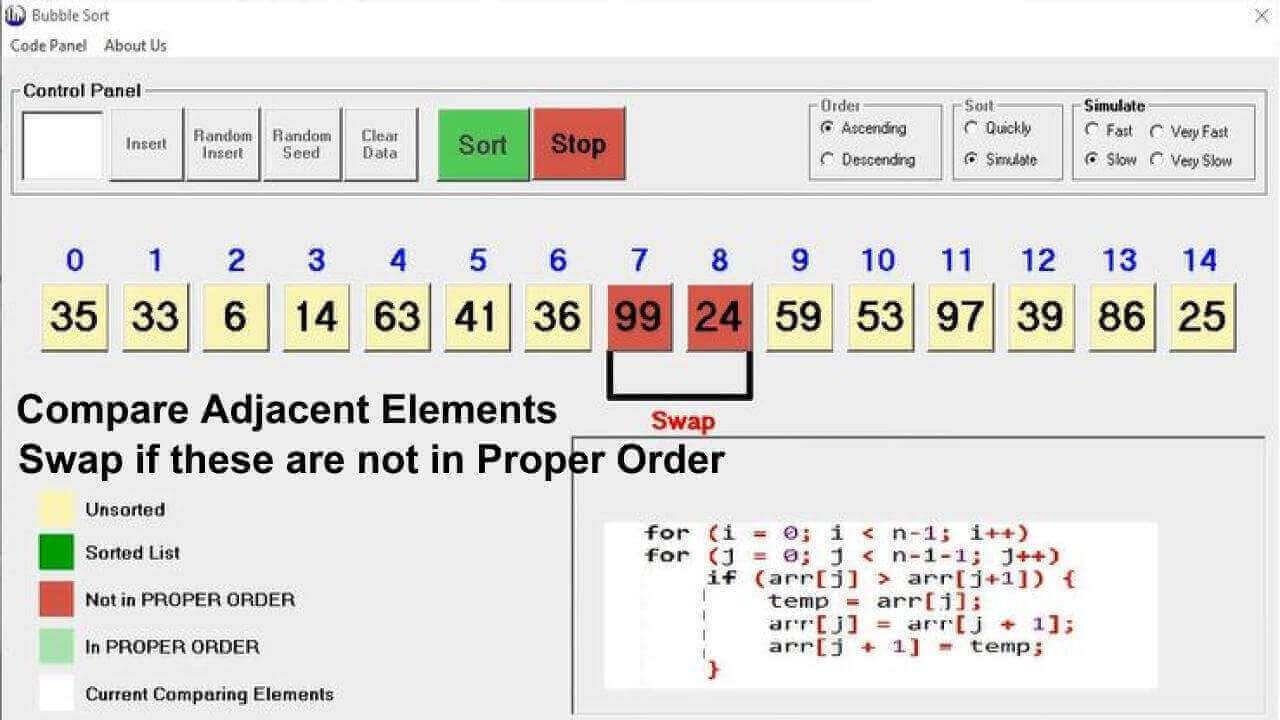# Bubble Sort

The simplest and basic sorting algorithm is known as bubble sort. Bubble sort algorithm implement basic sorting technique that involve comparison of adjacent values.Bubble sort algorithm is suitable only for small data sets. The complexity of bubble sort increases as the volumne of input data increases. Bubble sort is normally studied to understand the basic phenomenon of sorting,

## Algorithm

```Loop i = 0 To i < N Step 1
Loop j = 0 To N Step 1
If Array[ j ] > Array[ j + 1 ]
SWAP Array[ j ] , Array[ j + 1 ]
End If
Next j
Next i```

## Visual Presentation## Time complexity

 Sr Complexity Worst Case Average Case Best Case 1 Comparisons O(n)2 O(n)2 O(n) 2 Swaps O(n)2 O(n)2 O(1)

### Wrost Case:

When all the provided data items are not in proper order then this is considered as wrost case. In this situation both outter and inner loop (Nested Loop) will complete their iterations. And there will be swap in each iteration of inner loop.

### Average Case:

In average case there will be some data sorted in the list. This sorted data may be lie in any position eg: start, mid or last part of the array. But this will generate the similar situation for time complexity. Because bubble sort algorithm match/compare adjacent values. So that in average case there will the same time complexity as in worst case.

### Best Case:

In best case there will be constant time required for bubble sort.

## Space complexity

Bubble sort algorithm used only auxilary variables, So that space complexity is constant. In bubble sort algorithm we are required only counter variables and one temporary variable sometime named as temp for swapping data items that will not in proper order.

## Bubble sort visualization:

Visual basic animation is deveopled by binaryVisual. We can download visual presentation of bubble sort algorithm using this link.